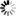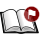Normal view

# Atomspheres of the components of close binary stars a thesis submitted to for the degree of doctor of philosophy in the faculty of Science, Bangalore University, Bangalore M. S. Rao [Ph.D Thesis]

Material type:TextPublication details: Bangalore Indian Institute of Astrophysics 2001Description: x, 178pSubject(s): Online resources: Dissertation note: Doctor of Philosophy Indian Institute of Astrophysics, Bangalore 2001 Summary: For theoretical modeling of binary systems one has to consider realistic models which takes into account the radiative transfer, hydrodynamics. reflection effect etc. Since the problem is complex, we study in the thesis some of idealized models which will help us in understanding the important physical processes in close binaries. Intially we have computed the theoretical lines in the expanding and extended distorted atmospheres of the components of close binary system. I have considered the necessary geometrical formalism for illumination of a stellar atmospheres from a source. We describe the method to calculate the radiation field from the irradiated surface of the component in a binary system. Chapter 1 Discrete Space theory of Radiative transfer: In this chapter a concise description of the lnethod of obtaining the solution of radiative transfer equation which can be applied to different geometrical and physical systellls is given. This method was developed by Grant and Peraiah (1972), and Peraiah and Grant(1973). This chapter deals with (1) interaction principle (2) star product (3) calculation of radiation field at internal points (4) integration of monochromatic radiative transfer equation and derivation of rand t operators of the" cell". (5) flux conservation and (6) line formation in expanding media. The radiative transfer equation in spherical symmetry is used for calculating the self radiation of the primary star in a binary system. Chapter 2 Reflection effect in close binaries : The aim of this section is to estimate the radiation field along the spherical surface of a primary component irradiated by an external point source of radiation. This can be applied to very widely separated systems. The transfer of radiation incident on the atmosphere of the component from the companion cannot be studied by using any symmetric solution of the equation of transfer. This needs a special treatment. We adopt angle-free one dimensional model (see Sobolev 1963). Chapter 3 Incident radiation from an extended source: The effects of irradiation from an extended source of the secondary cOInponent on the atmospheres of the primary are studied. Chapter 4 (1) Effects of reflection on spectral line forlllation: Effects of reflection on formation of spectral lines in a purely scattering atmosphere and studied how the equivalent widths change when irradiation from the secondary is taken into account. However, these calculations were done in static atnlospheres. So in the next step we have included the expanding atmospheres. (2) Effects of irradiation on the line formation in the expanding atmospheres of the components of a close binary system : We studied the formation of lines in the irradiated expanding atmospheres of the component of close binary system. We considered two-level atom approximation in non-LTE situation with complete l'<.'clistribution. We assumed that the dust scatters isotropically in the atmosphere. The line profiles of the dusty atmosphere are compared with those formed in dust free atmosphere. The profiles are presented for different velocities of expansion, proximity of secondary component to the primary, and dust optical depths. The line profiles for a dust free atmosphere with and without reflection effects are computed and compared. Chapter 5 Distorted surface due to self rotation and tidal forces: In this chapter a general expression for gravity darkening of the tidally uniformly rotating roche components of close binary system is derived. This theory is used to calculate the line profiles taking into account rotation and expansion velocities. Chnpter 6 Effect of gravity darkening on spectral line forlnation : We studied the transfer of line radiation in the atmospheres of close binary components whose atmospheres are distorted by the self radiation and tidal forces due to the presence of the secondary component. The distortion is measured in terms of the ratio of angular velocities at the equator and pole, mass ratio of the two components, the ratio of centrifugal force to that of gravity at the equator and the ratio of the equatorial radius to the distance between the centers of gravity. We obtain the equation of the distorted surface by solving a seventh degree equation which contains the above parameters. Transfer of line radiation is studied in such asymmetric atmosphere assuming complete redistribution and a two-level atom approximation. The atmosphere is assumed to be expanding radially. Various black body temperatures are being used to describe the total luminosity of the components for the purpose of irradiation. Chapter 7 Conclusions: We present important results obtained from this study of research from each chapter.
Tags from this library: No tags from this library for this title.
Star ratingsAverage rating: 0.0 (0 votes)
Holdings
Item type Current library Shelving location Call number Status Date due BarcodeThesis & Dissertations IIA Library-Bangalore General Stacks (043)524.38 (Browse shelf(Opens below)) Available 15094

Thesis Supervisor Prof. A. Peraiah

Doctor of Philosophy Indian Institute of Astrophysics, Bangalore 2001

For theoretical modeling of binary systems one has to consider realistic
models which takes into account the radiative transfer, hydrodynamics.
reflection effect etc. Since the problem is complex, we study in the thesis
some of idealized models which will help us in understanding the important
physical processes in close binaries. Intially we have computed the
theoretical lines in the expanding and extended distorted atmospheres of
the components of close binary system. I have considered the necessary
geometrical formalism for illumination of a stellar atmospheres from a
source. We describe the method to calculate the radiation field from the
irradiated surface of the component in a binary system.
Chapter 1
Discrete Space theory of Radiative transfer: In this chapter a
concise description of the lnethod of obtaining the solution of radiative
transfer equation which can be applied to different geometrical and physical
systellls is given. This method was developed by Grant and Peraiah
(1972), and Peraiah and Grant(1973). This chapter deals with (1) interaction
principle (2) star product (3) calculation of radiation field at
internal points (4) integration of monochromatic radiative transfer equation
and derivation of rand t operators of the" cell". (5) flux conservation
and (6) line formation in expanding media.
The radiative transfer equation in spherical symmetry is used for calculating
the self radiation of the primary star in a binary system.
Chapter 2
Reflection effect in close binaries : The aim of this section is to
estimate the radiation field along the spherical surface of a primary component
applied to very widely separated systems. The transfer of radiation incident
on the atmosphere of the component from the companion cannot be
studied by using any symmetric solution of the equation of transfer. This
needs a special treatment. We adopt angle-free one dimensional model
(see Sobolev 1963).
Chapter 3
from an extended source of the secondary cOInponent on the
atmospheres of the primary are studied.
Chapter 4
(1) Effects of reflection on spectral line forlllation: Effects of
reflection on formation of spectral lines in a purely scattering atmosphere
and studied how the equivalent widths change when irradiation from the
secondary is taken into account. However, these calculations were done
in static atnlospheres. So in the next step we have included the expanding
atmospheres.
(2) Effects of irradiation on the line formation in the expanding
atmospheres of the components of a close binary system : We
studied the formation of lines in the irradiated expanding atmospheres
of the component of close binary system. We considered two-level atom
approximation in non-LTE situation with complete l'<.'clistribution. We
assumed that the dust scatters isotropically in the atmosphere. The line
profiles of the dusty atmosphere are compared with those formed in dust
free atmosphere. The profiles are presented for different velocities of
expansion, proximity of secondary component to the primary, and dust
optical depths.
The line profiles for a dust free atmosphere with and without reflection
effects are computed and compared.
Chapter 5
Distorted surface due to self rotation and tidal forces: In this
chapter a general expression for gravity darkening of the tidally uniformly
rotating roche components of close binary system is derived. This theory
is used to calculate the line profiles taking into account rotation and
expansion velocities.
Chnpter 6
Effect of gravity darkening on spectral line forlnation : We studied
the transfer of line radiation in the atmospheres of close binary components
whose atmospheres are distorted by the self radiation and tidal
forces due to the presence of the secondary component. The distortion
is measured in terms of the ratio of angular velocities at the equator and
pole, mass ratio of the two components, the ratio of centrifugal force to
that of gravity at the equator and the ratio of the equatorial radius to the
distance between the centers of gravity. We obtain the equation of the
distorted surface by solving a seventh degree equation which contains the
above parameters. Transfer of line radiation is studied in such asymmetric
atmosphere assuming complete redistribution and a two-level atom
approximation. The atmosphere is assumed to be expanding radially.
Various black body temperatures are being used to describe the total
luminosity of the components for the purpose of irradiation.
Chapter 7
Conclusions: We present important results obtained from this study
of research from each chapter.

There are no comments on this title.